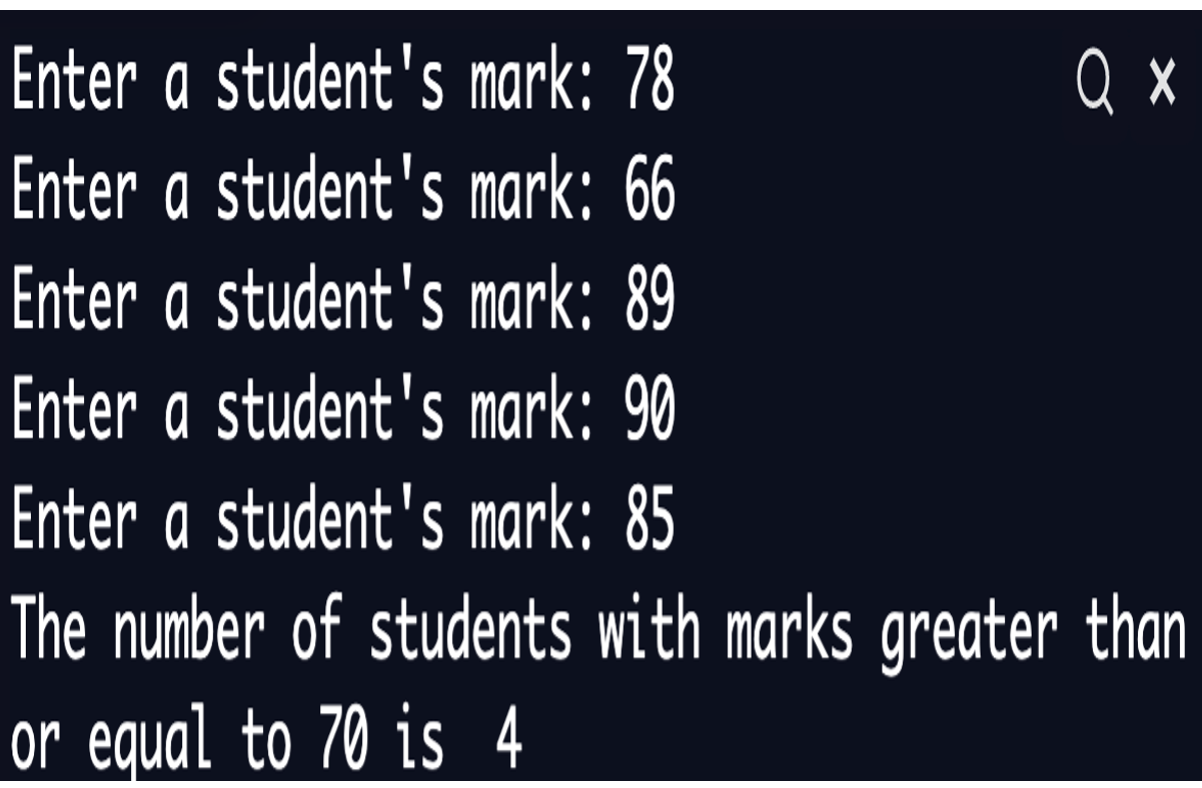# For Loops Exercise and Solution 7

Exercise 6: Write a program to read 5 students’ marks and find and display how many students with a mark greater than or equal to 70.

See below the code and output:
```
counter=0
for i in range (5):
Mark = float (input("Enter a student's mark: "))
if (Mark >= 70):
counter = counter+1
print ("The number of students with marks greater "+ "than or equal to 70 is ", counter)
```